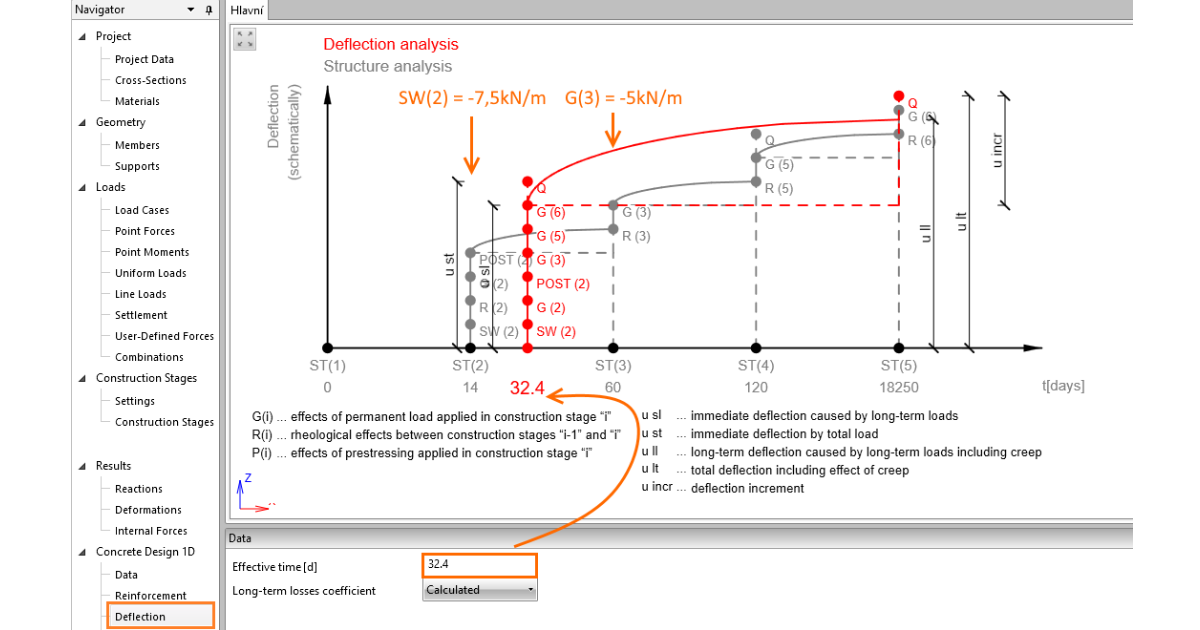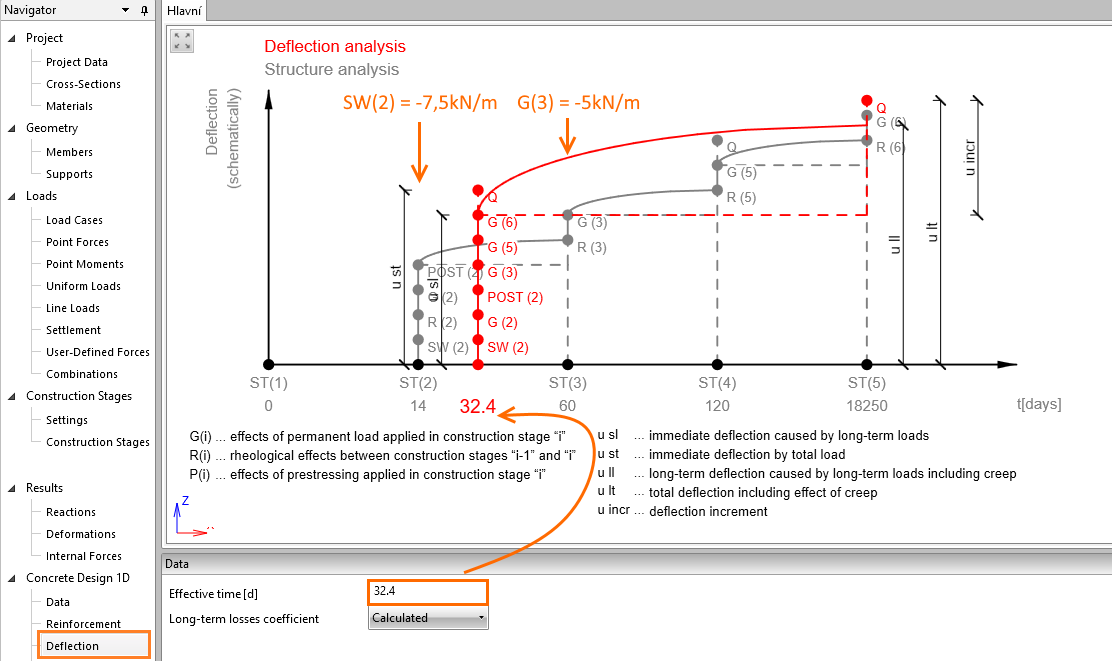### Choose language# How to set the effective time for analysis of deflection

$$The simplified method of effective time is used for the calculation and check of deflection. While setting the effective time value, the scheme of TDA analysis with increments of permanent and variable cases is shown in the Main window (grey lines). The red line represents an approximation curve of calculation of deflection according to given effective time value.This method merges the effects of all increments to the specified time and calculates all short-term and long-term deflections listed below the scheme. The method recommends inputting the effective time value to the time of application of the biggest portion of permanent loads or between the dates of application of two biggest permanent loads.The application of self-weight -7.5kN/m at 14 days and superimposed dead load -5 kN/m at 60 days will serve as an example. You will get to a time of 32.4 days by a simple distribution by load values.$$